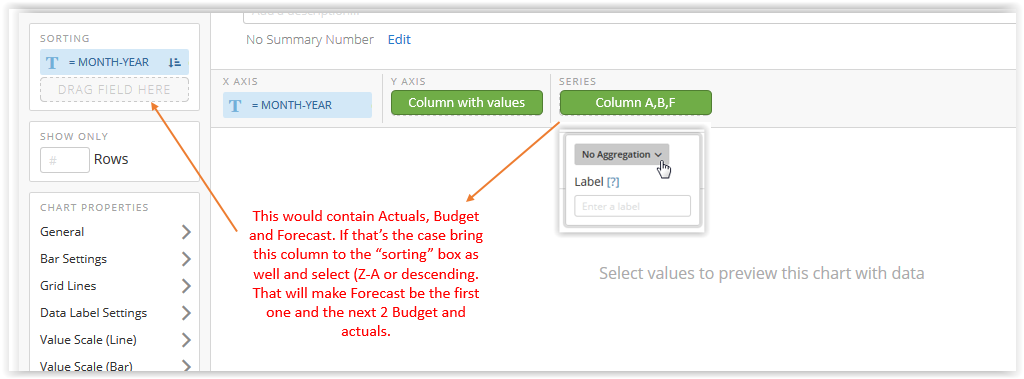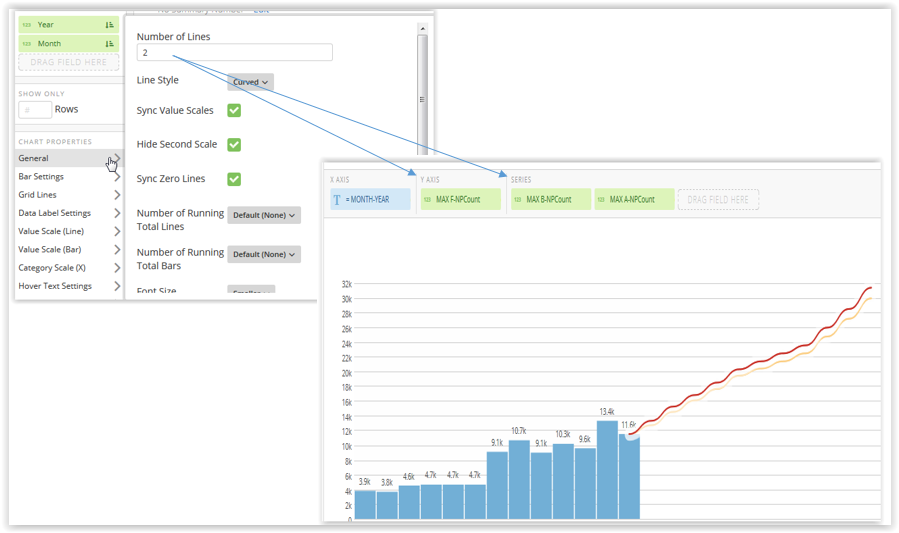Group bar and line chart

Can I assign each series to bars and lines as I want or will the program automatically decide? Let me know how to get this done.

I understand that I can decide how many line charts I want to see on the grouped bar chart but that doesn't seem to resolve the issue I am experiencing.

Thanks for any help with this!

• Ugh! thats a good question,

I think it can be tackled 2 different ways.

1) Use the sorting box when building your chart. follow this example belowIf it does not work then

2) create 3 beastmodes :

a) actuals = sum(case when 'Column ABF' = Actuals Then 'Column with values' End)

it could be count , average or any aggregation you need

b) Budget = same formula but  when 'Column ABF' = Budget

c) Forecast = same as above but when it equals Forecast

That will create 3 diferent series that you can bring into the chart in the order you prefer.

Give it a try and let me know if that works for you

• Yes you can, in order for it to work go to the "chart properties", Then under "General" you can specify the number of lines you want to see. Just make sure to position the series containing the values in first.

Take a look at the image below , it shows exactly what I mean